Next: Description of the program Up: Theoretical overview Previous: Phonon frequencies

## Calculation of the force constant matrix

We calculate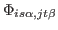by the small-displacement method. In harmonic approximation the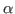Cartesian component of the force exerted on the atom at position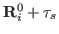is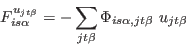(2)

where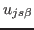is the displacement of the atom in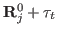along the direction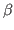and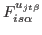is thecomponent of the force induced on the atom at position. The force constant matrix can be calculated as: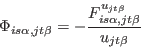(3)

by displacing once at a time all the atoms of the lattice along the three Cartesian components by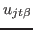, and calculating the forces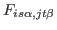induced on the atoms in. Eqn.( 3) computes the force constant matrix using forward differences; for numerical reasons, it can be advantageous in some cases to use central differences, in which case the force constant matrix can be calculated as: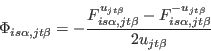(4)

Since the crystal is invariant under traslations of any lattice vector, it is only necessary to displace the atoms in one primitive cell and calculate the forces induced on all the other atoms of the crystal. In what follows we will assume this as understood and put simply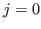.

It is important to appreciate that the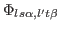in the formula for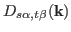is the force-constant matrix in the infinite lattice, with no restriction on the wavevector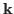, whereas the calculations ofcan only be done in supercell geometry. Without a further assumption, it is strictly impossible to extract the infinite-latticefrom supercell calculations, since the latter deliver information only at wavevectors that are reciprocal lattice vectors of the superlattice. The further assumption needed is that the infinite-latticevanishes when the separation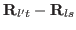is such that the positions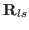and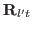lie in different Wigner-Seitz (WS) cells of the chosen superlattice. More precisely, if we take the WS cell centred on, then the infinite-lattice value ofvanishes ifis in a different WS cell; it is equal to the supercell value ifis wholly within the same WS cell; and it is equal to the supercell value divided by an integer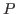iflies on the boundary of the same WS cell, whereis the number of WS cells havingon their boundary. With this assumption, theelements will converge to the correct infinite-lattice values as the dimensions of the supercell are systematically increased.

It is not always necessary to displace all the atoms in the primitive cell, since the use of symmetries can reduce the amount of work needed. This is done as follows. We displace one atom in the primitive cell, let's call it 'one', and we calculate the forces induced by the displacement on all the other atoms of the supercell. Then we pick up one other atom of the primitive cell, atom 'two'. If there is a symmetry operation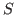(not necessarily a point group symmetry operation) such that, whenis applyed to the crystal atom two is sent into atom one and the whole crystal is invariant under such transformation, then it is not necessary to displace atom two, and the part of the force constant matrix associated with its displacement can be calculated using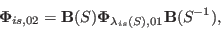(5)

where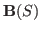is the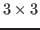matrix representing the point group part ofin Cartesian coordinates, and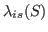indicates the atom of the crystal where the atom inis brought because of the action of the symmetry operation. If there is no symmetry operation connecting atom two to atom one then atom two is displaced and all the induced force field is calculated. The procedure is repeated for all the atoms of the primitive cell.

In principle each atom has to be displaced along the three Cartesian directions. It is sometimes convenient to displace the atoms along some special directions so as to maximize the number of symmetry operations still present in the 'excited' supercell, in this way the calculations of the forces are less expensive. This can always be done, as long as one displaces the atoms along three linearly independent directions. The forces induced by the displacements along the three Cartesian directions is easily reconstructed by the linear combination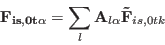(6)

where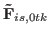is the force induced on the atom indue to a displacement of the atom in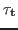along the direction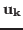, and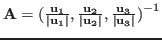is the inverse of thematrix whose columns are the normalized displacements in Cartesian coordinates.

Using symmetries it is possible to reduce the number of displacements even further: if applying a point group symmetry operation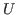to the displacement vector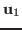one obtains a vector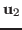which is linearly independent from, then the force field that would be induced by the displacementcan be calculated by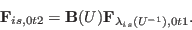(7)

If a linearly independent direction cannot be found one has to displace the atom along a chosen independent direction and perform an other calculation. This is done untill a set of three independent directions is found.

The force constant matrix is invariant under the point group symmetry operations of the crystal. This is not automatically garanteed by the procedure just described, because in general the crystal is not harmonic, and therefore eqns.( 34) are only an approximation. So, the force constant matrix must be symmetrized with respect to the point group operations of the crystal: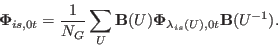(8)

The symmetrization of the force constant matrix removes all even-order anharmonicities . The harmonic approximation becomes better and better as the displacement are made smaller and smaller. However, if the displacements are small, also the force induced are small, but there is a limit in the accuracy achievable in the calculations, so one cannot make too small displacements. Usually a fraction of a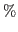of the nearest-neighbour distance is a good compromise.

As an example of the procedure just described let's consider the h.c.p. crystal. There are two atoms in the primitive cell, so in principles we would need six independent calculations. We will see that the number of calculations needed is equal to two. In first place one can easily recognize that only one atom needs to be displaced: if we traslate the crystal from one atom to the other and we perform a spatial inversion the crystal remains unchanged. Secondly, by applying a clockwise rotation ofdegrees, for example, to a displacement in the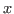direction, one obtains an independent displacement. So only one additional displacement along the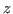direction is needed.Next: Description of the program Up: Theoretical overview Previous: Phonon frequencies
Dario Alfe` 2012-02-20